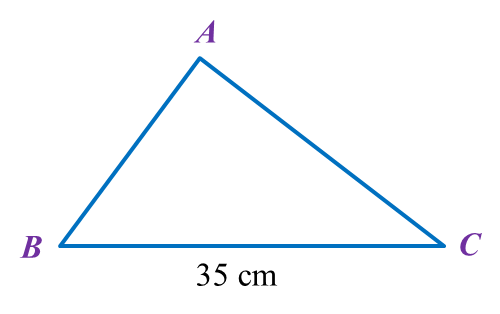# 4.2.2 Ratio, Rates and Proportions, PT3 Focus Practice

Question 5:
In diagram below, ABC is a triangle.The sides of the triangle are in the ratio AB : BC: CA = 4 : 7 : 6.
Find the difference in length, in cm, between AB and CA.

Solution:
$\begin{array}{l}AB:BC:CA=4:7:6\\ BC=35cm\\ \\ \frac{AB}{BC}=\frac{4}{7}\\ \frac{AB}{35}=\frac{4}{7}\\ AB=\frac{4}{7}×35\\ \text{}=20cm\\ \frac{CA}{35}=\frac{6}{7}\\ CA=\frac{6}{7}×35\\ \text{}=30cm\end{array}$

Difference in length between AB and CA
= 30 – 20
= 10 cm

Question 6:
In a campaign of selling T shirt for Merdeka Day makes an amount of profit, RM x. The profit is divided between Jamal and Rafizi in the ratio of 5 : 3. Jamal receives RM 5400 more than Rafizi.

Calculate the value of x.

Solution:
Portion of profit for Jamal = ⅝
Portion of profit for Rafizi = ⅜
Given Jamal receives RM 5400 more than Rafizi,

Question 7:
Karim and Roger share RM300 in the ratio of 2 : 3. Karim gives ⅓ of his share to Mandeep. Then Mandeep received RM60 from Roger.

Find the ratio of Karim’s money to roger’s money to Mandeep’s money.

Solution: